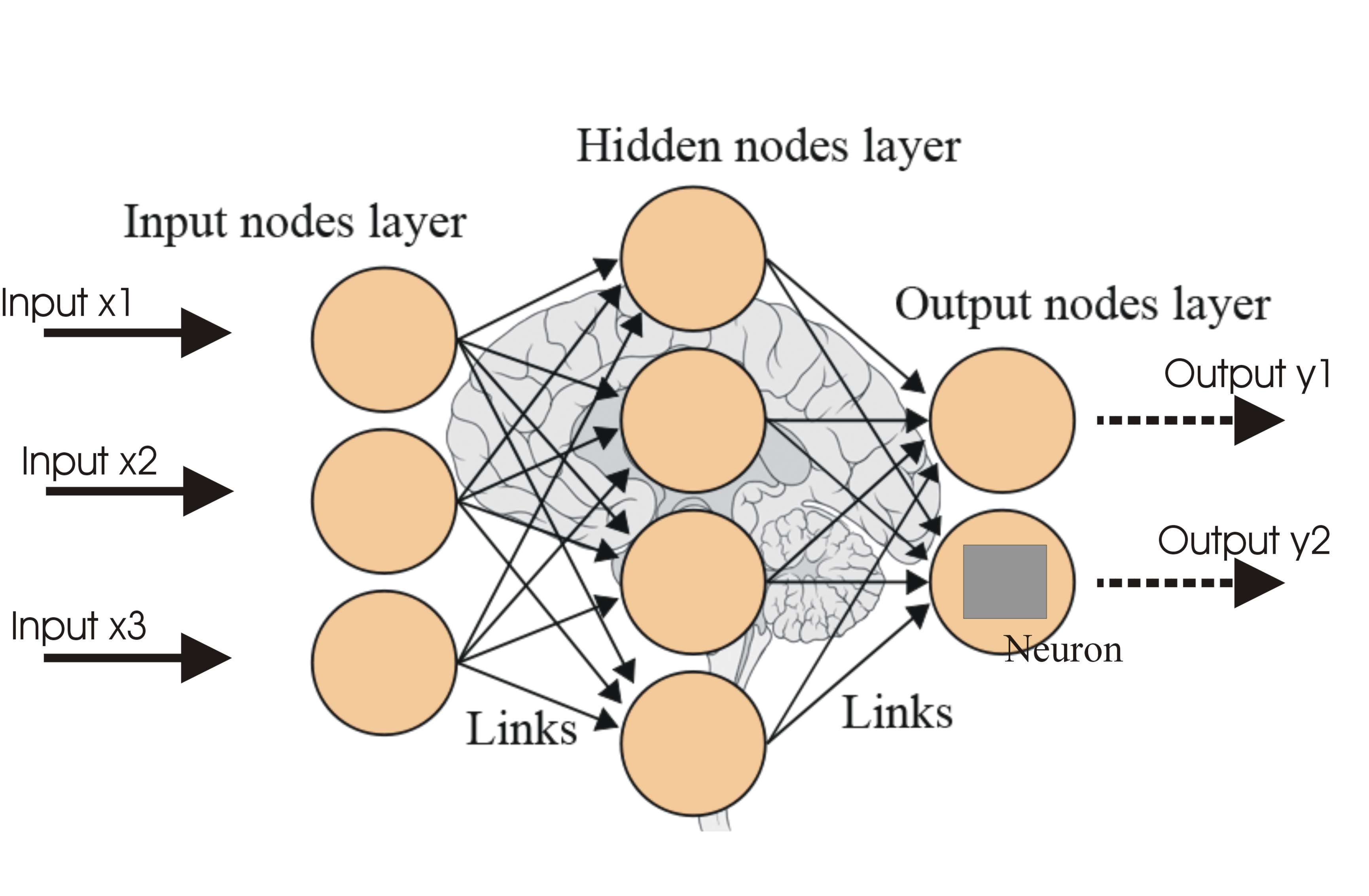A neural network is a branch of machine learning which operates like a human brain. This generates an artificial neural network with an algorithm that helps the machine to learn by generating new data. Whereas machine learning is a sub-field of Artificial Intelligence that helps the algorithm to learn and advance automatically from experience without being directly programmed. In machine learning, functions are divided into various groups, and neural networks construct algorithm models using neurons for machine learning, and this is how to distinguish between neural networks vs machine learning. Below is the difference between machine learning and neural networks.### Difference Between Machine learning and Neural Networks

A neural network is a type of machine learning algorithm that is typically used in supervised learning. However, the neural network is a group of algorithms for machine learning. Machine learning models will follow the function which is learned from the data and at some point, it still needs more guidance. But in the case of neural networks algorithms are capable of making their decisions on their own. So, they are skills that have many differences between them. In machine learning, you need to knowledge in programming languages like Python/C++/R/Java then you would find a job in machine learning where C++ will help in speeding code, R works in statistics and plots and Hadoop is Java. Even you need to learn Probability and Statistics like Naïve Bayes, Gaussian mixture models, and hidden Markov models which can help in understanding these models. Also, data modeling and Evaluation is part of machine learning that can help to choose the appropriate accuracy.But the neural networks require skills of Artificial Intelligence which can be acquired through training courses and actual field experience. The skills you require need to have problem-solving and math skills and also a deep understanding of algorithms and logical sense behind it. You need to have the ability to search for patterns from large amounts of data from able to draw conclusions from it.  By differentiating between neural networks vs machine learning, then you should say that both are equal in terms of programming, capabilities, and outcome.

Neural Networks and machine learning will fall under the same category of Artificial Intelligence, but neural networks are a subfield of machine learning while learning how to differentiate between neural networks vs machine learning. So, the machine learning algorithm will give us the results of what it has learned and neural networks will under the category of deep learning which powers the most human-like intelligence artificially. Hope that I have covered all the topics in my article about the difference between machine learning and neural networks. Thanks for reading!